# Difference between revisions of "Deformation, isometric"

of a submanifoldin a Riemannian spaceA deformation under which the lengths of curves inare unchanged. The problem of isometric deformation of surfaces originated with C.F. Gauss, and is a fundamental problem in differential geometry.

Apart from the general case, isometric deformations of surfaces preserving some external characteristic have been fairly thoroughly investigated; it usually turned out that only surfaces of a definite class allow of such deformations. Such are, for example: 1) surfaces where the mean curvature is preserved under isometric deformation (surfaces of constant mean curvature, and, in particular, minimal surfaces and some surfaces applicable on a surface of revolution); 2) surfaces where some family of asymptotic lines is preserved under isometric deformation (the ruled surfaces (cf. Ruled surface) are comprised in this class); 3) surfaces allowing a deformation over a principal base; etc.

In character, the problem of isometric deformation of surfaces in Euclidean space can be divided conventionally into that of local isometric deformation, that is, deformation of some small neighbourhood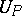of a pointin the surface, and global isometric deformation, that is, deformation of a pre-given piece of the surface or of the whole of it. As a rule, every surface allows of local isometric deformation; however, ifis a flat point, then under certain conditions and with strong regularity assumptions on the allowed deformation,turns out not to have an isometric deformation, even locally.

The first assertions on global isometric deformation were a theorem of E. Liebmann on the non-isometric deformability of the sphere, and also the following result of D. Hilbert: The maximum principal curvature radius of part of a surface of constant curvature 1 is attained on the boundary. In later investigations it was established that every closed convex surface is not isometrically deformable in the class of convex surfaces without additional regularity conditions. Theorems were discovered of maximum-principle type, relating to isometric deformations of an arbitrary piece of a convex surface of class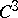, which imply that a closed convex surface is not isometrically deformable. However, it was proved that a sphere of classhas an isometric deformation in the same class.

Only partial results have been established concerning isometric deformation of closed surfaces of positive genus; for example, surfaces of type(tori), that is, surfaces consisting of a finite number of regions with piecewise-smooth boundaries in each of which the Gaussian curvaturedoes not change sign and vanishes only on the boundary, and the total curvature of all the regions with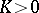is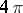, are not isometrically deformable under certain additional assumptions (analyticity, absence of two closed asymptotic lines); in particular, the ordinary torus is not isometrically deformable.

Complete non-closed surfaces are usually isometrically deformable. A complete convex surfaceis not isometrically deformable if, and is isometrically deformable if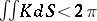; every admissible isometric deformation of it is determined by an isometric deformation of its limiting cone. Certain classes of complete surfaces of negative curvature also allow of an isometric deformation; for example, minimal surfaces, in particular a catenoid, can be isometrically deformed into a helicoid.

The study of isometric deformations of non-closed surfaces with boundary also relates mainly to surfaces with Gaussian curvature of constant sign, and the case where the isometric deformation is determined uniquely by different kinds of boundary conditions has been studied in detail. Thus, for any isometric deformation of a closed surface, at least one point of its boundarychanges its distance from a fixed point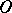from whichis viewed outwards and which is situated outside the convex hull (so thatis not isometrically deformable if the distance of an arbitrary point of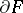fromis fixed); likewise, for any isometric deformation of a convex surfacethat is uniquely projectable onto a planeand bounded by a plane contour, at least one point ofchanges its distance from(so thathas no sliding isometric deformation, that is, an isometric deformation in which the points of the boundary move parallel to some plane); for an isometric deformation of a surface of this type, the curvatureofchanges for at least one point, and the number of alterations of sign of the difference of the curvatures on the boundaries ofand its deformation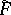is not less than four (so that if the curvature of the boundary is fixed,is not isometrically deformable). Concerning surfaces of negative curvature, a fairly wide class of isometric deformations of them are obtained from solutions of the Cauchy problem for the equations of Gauss and Peterson–Codazzi; for example, the isometric deformation of the piece of a surface with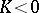bounded by segmentsof geodesics and two segments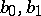of their orthogonal trajectories is uniquely determined by the isometric deformation of the boundary of. As for isometric deformations of surfaces with curvature of changing sign, results have been obtained only for the surfaces of revolution.

In the study of isometric deformation of surfaces, methods of the theory of partial differential equations are applied; for example, equations of elliptic type, which define isometric deformations of surfaces of positive Gaussian curvature, and also of surfaces for which the mean curvature is preserved; moreover, for convex surfaces, a special part is played by the method of direct limit transition of sequences of polytopes (see Glueing method) in combination with theorems on the regularity of a surface having a regular metric. When the convex surface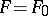is sufficiently close to its isometric deformation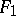, then the investigation of isometric deformations can be reduced by using a Cohn-Vossen transformation to analogous problems in the theory of infinitesimal deformations (cf. Infinitesimal deformation) of the so-called mean surface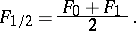How to Cite This Entry:
Deformation, isometric. Encyclopedia of Mathematics. URL: http://encyclopediaofmath.org/index.php?title=Deformation,_isometric&oldid=16801
This article was adapted from an original article by M.I. Voitsekhovskii (originator), which appeared in Encyclopedia of Mathematics - ISBN 1402006098. See original article